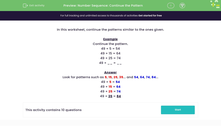# Number Sequence: Continue the Pattern

In this worksheet, students continue a sequence of numbers by working out the pattern.Key stage:  KS 2

Curriculum topic:   Number: Number and Place Value

Curriculum subtopic:   Solve Number Problems to 1000

Popular topics:   Ordering Numbers worksheets

Difficulty level:#### Worksheet Overview

In this activity, we will learn to continue the patterns in addition calculations.

In this pattern, the numbers get larger each time.

23 + 10 = 33

23 + 20 = 43

23 + 30 = 53

23 + 40 = 63

Did you notice that only the tens digit changes?

This is because a multiple of ten is added to 23 each time.

Let's try an example together.

See if you can spot the pattern and complete the last calculation.

Example

Continue the pattern.

49 + 5 = 54

49 + 15 = 64

49 + 25 = 74

49 + __ = __

If we look carefully, we can see two number patterns:

49 + 5 = 54

49 + 15 = 64

49 + 25 = 74

49 + __ = __

The number added to 49 increases by 10 each time.

The total also increases by 10 each time.

The two missing numbers are 35 and 84.

The final calculation can be completed as follows:

49 + 35 = 84

Now you have seen some number patterns in action, you are ready to try some questions on your own.

### What is EdPlace?

We're your National Curriculum aligned online education content provider helping each child succeed in English, maths and science from year 1 to GCSE. With an EdPlace account you’ll be able to track and measure progress, helping each child achieve their best. We build confidence and attainment by personalising each child’s learning at a level that suits them.

Get started